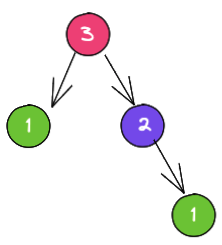# Recursion

September 15, 2021

TypeScript

Recursion is when a function calls itself(as a subroutine) until it can't, uhnnn.. A function to be considered recursive, it needs to follow two properties:

• It has to have a `base case` which will make it stop running infinitely
• It has to have a `recursive case` which will make it call itself until it reaches the `base case`

When we invoke functions, they get added to the Stack and once they're done running, they get removed from it. So if a recursive function doesn't have both properties, it'll be added to the Stack infinitely causing the Stack to overflow.

Here's the most famous recursive example: The Fibonacci sequece In the Fibonacci sequence each number is the sum of the two preceding ones. Say we want to calculate the Fibonacci of 3:

``````F(3) = F(1) + F(2)
F(1) = 1
F(2) = F(1) + F(0)
F(1) = 1
F(0) = 0``````

However, after reaching thee bottom, we add together every resulting number from the F function, for F(3) it is: 0 + 1 + 1 = 2, so F(3) = 2

Representing this as a tree it would look like this:Identifying the properties from a recursive function: If we look closer, the bottom case will always be 0 or 1, so:

• `base case` identifies as: F(0) = 0 or F(1) = 1
• `recursive case` would then be n > 1: F(n) = F(n - 1) + F(n - 2)
``````let fib = (n: number): number => {
if (n < 2) return n;
return fib(n - 1) + fib(n - 2);
}``````

Let's make one more recursive function, this time let's reverse an array of strings:

``````let str  = ['o', 't', 's', 'u', 'a', 'F'];
let reverse = ([head, ...tail]: string[]): string[] => {
}``````

Let's analyze the above algorithm and identify the properties of a recursive function:

The way this algorithm works it that:

• It destructures the input array and takes the first element and adds it into a stack.
• Then it calls itself with all the array elements but the first one.

So, our `base case` happens when we call the function with an empty array and the `recursive case` happens when we do call the function with an array of elements.

## Tail-Recursion

Tail call is a call performed as the final action of a procedure(source). If you take a look at the Fibonacci algorithm, you can see that the `recursive case` happens at the end of the function body:

``````let fib = (n: number): number => {
if (n < 2) return n;
return fib(n - 1) + fib(n - 2);
}``````

Not every recursive function is or needs to be tail-recursive, in the example above, we coul omit the return and store the result of the recursive call into a variable and do more stuff after that if we wanted to and then return whatever we wanted to.